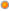# Q: I have a field in the pivot that shows duration on some event in seconds. How can I create calculated measure using DAX that would convert numeric result (seconds) to string in the format hh:mm:ss?

User Rating: 5 / 5Q: I have a field in the pivot that shows duration on some event in seconds. How can I create calculated measure using DAX that would convert numeric result (seconds) to string in the format hh:mm:ss?

For example, when my field value is 635, I would like to see 10:35 (that is 10min and 35 second) as this would be much easier to understand. How can I do this with DAX?

A: Lets say we have a field FactTable[DurationInSec] that contains duration of some events. We can create new calculated measure [Duration String] that will take value of measure [DurationInSec] and will translate that value to the string format hh:mm:ss. Here is how we will calculate values for hours, minutes and seconds

- seconds: we will divide [DurationInSec] by 60 and will use a reminder. Formula would be: MOD(Calculate(SUM(FactTable[DurationInSec])),60). As we would like to have leading zero for single digit durations, we will adjust formula: RIGHT("0" & MOD(Calculate(SUM(FactTable[DurationInSec])),60),2). Here x = [Duration in Sec]

- minutes: we will divide [DurationInSec] by 60*60 and will use reminder as a resulting number of minutes. Formula for that would be: FLOOR(MOD(Calculate(SUM(FactTable[DurationInSec])),3600)/60,1). As we do not want to show minutes part in our result if value of [DurationInSec] is less than 60seconds, so we will adjust minute formula to: IF(Calculate(SUM(FactTable[DurationInSec]))<60,"", RIGHT("0"&FLOOR(MOD(Calculate(SUM(FactTable[DurationInSec])),3600)/60,1),2))

- hours: we will divide [DurationInSec] by 3600 (60*60) and will use whole integer part. Formula for that would be: FLOOR(Calculate(SUM(FactTable[DurationInSec]))/60/60,1). We will not show hours part of the string if duration is less than 1 hour, so adjusted hour formula would be: IF(Calculate(SUM(FactTable[DurationInSec]))<60*60,"",FLOOR(Calculate(SUM(FactTable[DurationInSec]))/60/60,1) & ":").

So our final DAX formula would be:

IF(Calculate(SUM(FactTable[DurationInSec]))<60*60,"",FLOOR(Calculate(SUM(FactTable[DurationInSec]))/60/60,1) & ":") & IF(Calculate(SUM(FactTable[DurationInSec]))<60,"", RIGHT("0"&FLOOR(MOD(Calculate(SUM(FactTable[DurationInSec])),3600)/60,1),2)) & ":" & RIGHT("0" & MOD(Calculate(SUM(FactTable[DurationInSec])),60),2)

Here are samples of results:

 Duration in Sec Duration String 7 :07 17 :17 67 01:07 77 01:17 607 10:07 3607 1:00:07 3677 1:01:17

Note: It would be also very easy to adjust this formula to disply results like 1h 17min 27sec.

Tags: dax, faq, excel

2007-2015 VidasSoft Systems Inc.